Linear Programming - Meaning & Definition

Linear programming is a technique to get the best outcome (for example maximum profit or minimum cost) in a mathematical model whose requirements are shown by linear relationships.

Linear programming (LP) is actually a special case of mathematical optimization.

The word 'linear' means that the relationships are represented by straight lines, i.e., the relationships are of the form k = p + qx. In other words, it is used to describe the relationships among two or more variables, which are directly proportional. The word 'programming' means the optimal allocation of limited resources.

In fact, every organization faces the problem of allocating limited resources to different activities. Such type of problem arises when there are alternative ways of performing a number of activities. For instance, consider a manufacturing firm where it is possible to manufacture a variety of products. Each of the products has a certain margin of profit per unit. These products use a common pool of resources whose availability is limited. Now the problem is to carefully allocate these resources to different types of finished products in such a way so that the total return may be maximum. In such a situation, the management's decision may be based on past experience and intuition, but decision so made is subjective rather than objective.

"Telling the future by looking at the past assumes that conditions remain constant. This is like driving a car by looking in the rearview mirror." -Herb Brody

Definition of Linear Programming

Linear Programming (LP) is a versatile technique for assigning a fixed amount of resources among competing factors, in such a way that some objective is optimized and other defined conditions are also satisfied.

Linear programming is a mathematical technique for determining the optimal allocation of resources and obtaining a particular objective when there are alternative uses of the resources. The objective may be cost minimization or inversely profit maximization.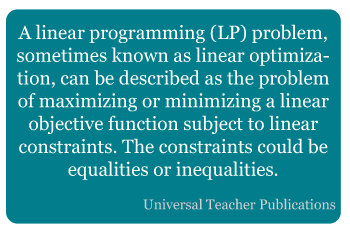Linear programming has been successfully applied to a variety of problems of management, such as production, advertising, transportation, refinery operation, investment analysis, etc. Over the years, linear programming has been found useful not only in business and industry but also in non-profit organizations such as government, hospitals, libraries, education, etc. Actually, linear programming improves the quality of decisions by amplifying the analytic abilities of a decision maker.

Please note that the result of the mathematical models that you will study cannot substitute for the decision maker's experience and intuition, but they provide the comprehensive data needed to apply his knowledge effectively.

"Experience is a comb which nature gives to men when they are bald." -Anonymous

Share and Recommend

•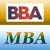MBA & BBA
•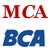BCA & MCA
•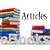Ebooks & Articles
•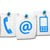Contact us